## 功能介紹

1. 取得當下時間

• js

``````new Date()
``````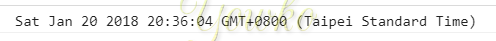• c#

``````DateTime.Now
``````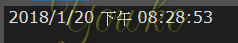• moment.js

``````moment().format()
``````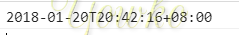2. 時間格式轉換

‘2018/01/01 00:00:00’

• js

``````var now = new Date();
var year = now.getFullYear();
var month = ("0" + (now.getMonth() + 1)).slice(-2);
var day = ("0" + (now.getDate() + 1)).slice(-2);
var hour = ("0" + (now.getHours() )).slice(-2);
var minute = ("0" + now.getMinutes() ).slice(-2);
var second = ("0" + now.getSeconds() ).slice(-2);
year + '/' + month + '/' + day + ' ' + hour + ':' + minute+ ':' + second
``````
• c#

``````DateTime.Now.ToString("yyyy/MM/dd HH:mm:ss")
``````
• moment.js

``````moment().format('YYYY/MM/DD HH:mm:ss')
``````
3. 時間處理

加一天

• js

``````var now = new Date();
var year = now.getFullYear();
var month = ("0" + (now.getMonth())).slice(-2);
var day = ("0" + (now.getDate() + 1)).slice(-2);
var hour = ("0" + (now.getHours())).slice(-2);
var minute = ("0" + now.getMinutes()).slice(-2);
var second = ("0" + now.getSeconds()).slice(-2);
new Date(year, month, day, hour, minute, second);
``````
• c#

``````DateTime.Now.AddDays(1)
``````
• moment.js

``````moment().add(7, 'days');
``````
``````moment().add(7, 'd');
``````

KeyShorthand
yearsy
quartersQ
monthsM
weeksw
daysd
hoursh
minutesm
secondss
millisecondsms
4. 取得當天開頭時間及結束時間

• js

請原諒小弟 js 略過, 個人能力不足寫不出來

• c#

``````DateTime.Today.ToString("yyyy/MM/dd HH:mm:ss");
``````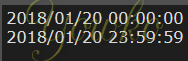• moment.js

``````moment().startOf('day').format('YYYY/MM/DD HH:mm:ss')
moment().endOf('day').format('YYYY/MM/DD HH:mm:ss')
``````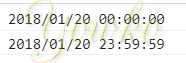## 心得

moment.js 功能很多，使用上很方便，最重要的是文件算是滿完整的，讓實際導入時的問題少很多，至於其他功能：指定時間、顯示格式、時間比較、多語言…各式操作就不一一紀錄，請直接參考 Moment.js